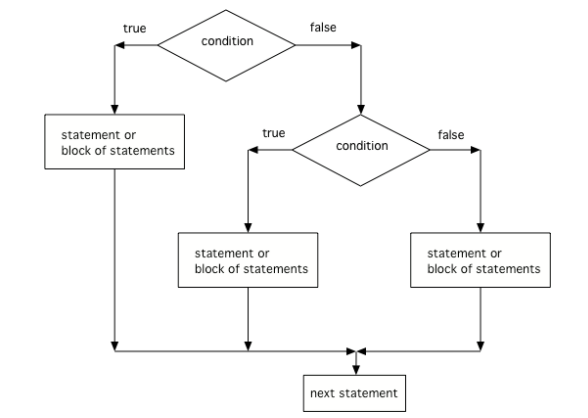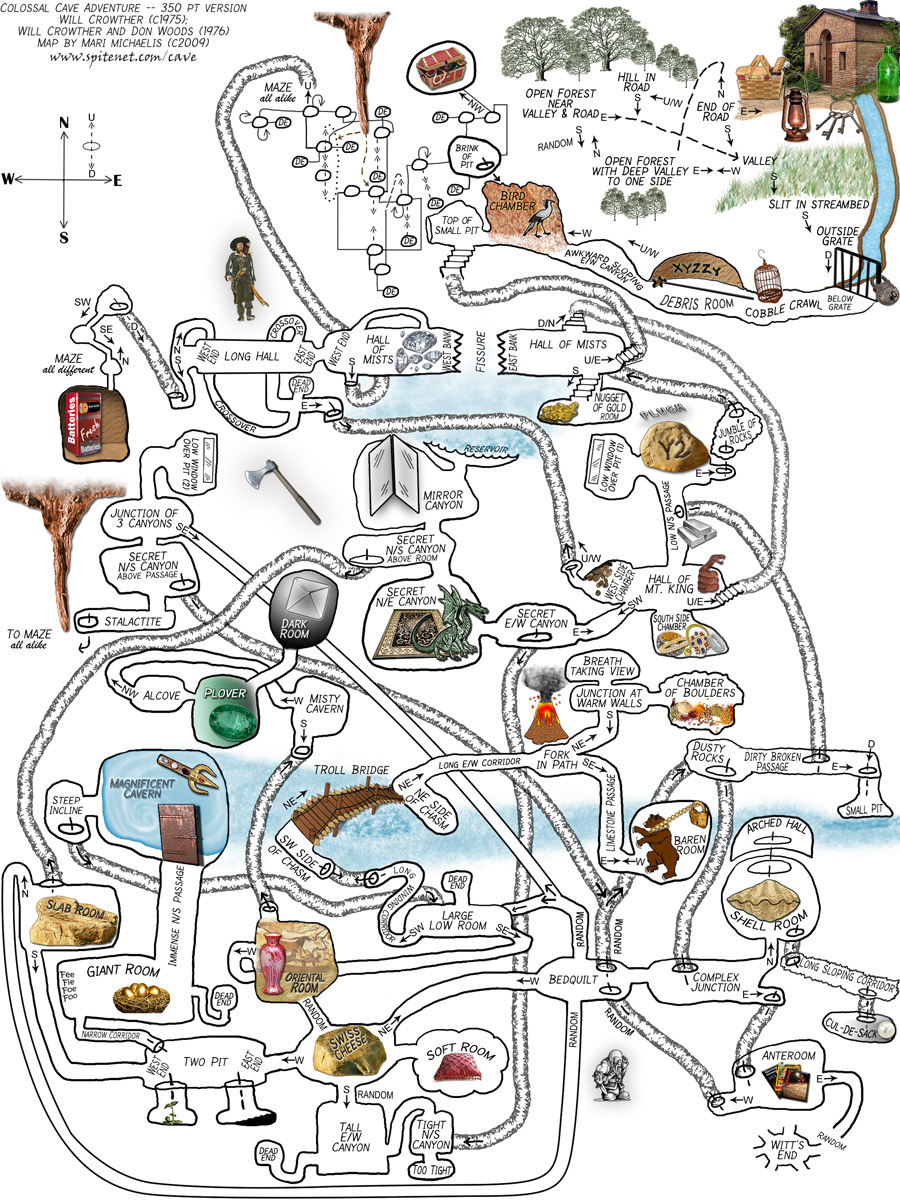# 3.4. Multi-Selection: else-if Statements¶

Using if/else statements, you can even pick between 3 or more possibilites. Just add else if for each possibility after the first if, and else before the last possibility.

  1 2 3 4 5 6 7 8 9 10 11 12 13 // 3 way choice with else if if (boolean expression) { statement1; } else if (boolean expression) { statement2; } else { statement3; } 

Run the code below and try changing the value of x to get each of the three possible lines in the conditional to print.

Here is a flowchart for a conditional with 3 options like in the code above.Figure 1: The order that statements execute in a conditional with 3 options: if, else if, and else

Note

Another way to handle 3 or more conditional cases is to use the switch and break keywords, but these will not be on the exam. For a tutorial on using switch see https://docs.oracle.com/javase/tutorial/java/nutsandbolts/switch.html.Check your understanding

3-4-1: What does the following code print when x has been set to -5?

  1 2 3 4 5 6 7 8 9 10 11 12 if (x < 0) { System.out.println("x is negative"); } else if (x == 0) { System.out.println("x is zero"); } else { System.out.println("x is positive"); } 
• x is negative
• When x is equal to -5 the condition of x < 0 is true.
• x is zero
• This will only print if x has been set to 0. Has it?
• x is positive
• This will only print if x is greater than zero. Is it?

3-4-2: What does the following code print when x has been set to 2000?

  1 2 3 4 5 6 7 8 9 10 11 12 if (x < 0) { System.out.println("x is negative"); } else if (x == 0) { System.out.println("x is zero"); } else { System.out.println("x is positive"); } 
• x is negative
• This will only print if x has been set to a number less than zero. Has it?
• x is zero
• This will only print if x has been set to 0. Has it?
• x is positive
• The first condition is false and x is not equal to zero so the else will execute.

3-4-3: What does the following code print when x has been set to .8?

  1 2 3 4 5 6 7 8 9 10 11 12 13 14 15 16 if (x < .25) { System.out.println("first quartile"); } else if (x < .5) { System.out.println("second quartile"); } else if (x < .75) { System.out.println("third quartile"); } else { System.out.println("fourth quartile"); } 
• first quartile
• This will only print if x is less than 0.25.
• second quartile
• This will only print if x is greater than or equal to 0.25 and less than 0.5.
• third quartile
• The first only print if x is greater than or equal to 0.5 and less than 0.75.
• fourth quartile
• This will print whenever x is greater than or equal to 0.75.

The else-if connection is necessary if you want to hook up conditionals together. In the following code, there are 4 separate if statements instead of the if-else-if pattern. Will this code print out the correct grade? First, trace through the code to see why it prints out the incorrect grade. Then, fix the code by adding in 3 else’s to connect the if statements and see if it works.

Finish the following code so that it prints “Plug in your phone!” if the battery is below 50, “Unplug your phone!” if it is above 100, and “All okay!” otherwise. Change the battery value to test all 3 conditions.

## 3.4.1.Programming Challenge : Adventure¶We encourage you to work in pairs for this challenge which is on repl.it (you will need an account there if you want to save your version).

One of the first games coded for early computers in the 1970s was called Colossal Cave Adventure. It was a text-based interactive fiction game where you had to make your way through an elaborate cave. The program only understood one word or phrase commands like north, south, enter, take, etc. You can try playing Adventure recreated online following some of the commands in this walkthrough. Part of the challenge is finding the commands that the code will understand.

In a game like Adventure, else if statements can be used to respond to commands from the user like n, s, e, w.

1. Try the program below or with this repl link. This is a very simple adventure game that lets the user move in 4 different directions. Right now, it only lets the user move north.

2. Add in else if statements to go in the directions of “s” for south and “e” for east, and an else statement that says “You can’t go in that direction”. Be creative and come up with different situations in each direction.

1. How many test-cases are needed to test all branches of your code?

2. If your class has time, your teacher may ask you to expand this game further or to come up with a different adventure location.

3-4-4: After you complete your code on repl, paste in a link to it (click on share) here.

## 3.4.2. Summary¶

• A multi-way selection is written when there are a series of conditions with different statements for each condition.

• Multi-way selection is performed using if-else-if statements such that exactly one section of code is executed based on the first condition that evaluates to true.

  1 2 3 4 5 6 7 8 9 10 11 12 13 // 3 way choice with else if if (boolean expression) { statement1; } else if (boolean expression) { statement2; } else { statement3; }